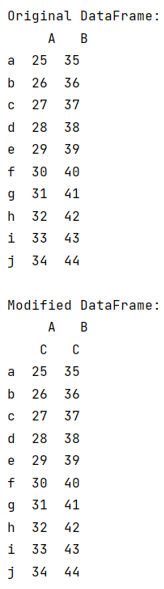# How to simply add a column level to a pandas dataframe?

Given a Pandas DataFrame, we have to simply add a column level to a pandas dataframe.
Submitted by Pranit Sharma, on July 23, 2022

Pandas is a special tool that allows us to perform complex manipulations of data effectively and efficiently. Inside pandas, we mostly deal with a dataset in the form of DataFrame. DataFrames are 2-dimensional data structures in pandas. DataFrames consist of rows, columns, and data.

Columns are the different fields that contains their particular values when we create a DataFrame. We can perform certain operations on both rows & column values. In this article, we are going to learn how to drop a level from a multi-level column index.

Multilevel indexing is a type of indexing that include different levels of indexes or simply multiple indexes. The DataFrame is classified under multiple indexes and the topmost index layer is presented as level 0 of the multilevel index followed by level 1, level 2, and so on.

## Add a column level to a pandas dataframe

To simply add a column level to a pandas DataFrame, we will first create a DataFrame then we will append a column in DataFrame by assigning df.columns to the following code snippet:

## Syntax

```pd.MultiIndex.from_product([df.columns, ['Col_name']])
```

Let us understand with the help of an example,

## Python program to simply add a column level to a pandas dataframe

```# Importing pandas package
import random

import pandas as pd

# Creating a Dictionary
d={
'A':[i for i in range(25,35)],
'B':[i for i in range(35,45)]
}

# Creating a DataFrame
df = pd.DataFrame(d,index=['a','b','c','d','e','f','g','h','i','j'])

# Display original DataFrame
print("Original DataFrame:\n",df,"\n")

df.columns = pd.MultiIndex.from_product([df.columns, ['C']])

# Display modified DataFrame
print("Modified DataFrame:\n",df)
```

## Output

The output of the above program is: